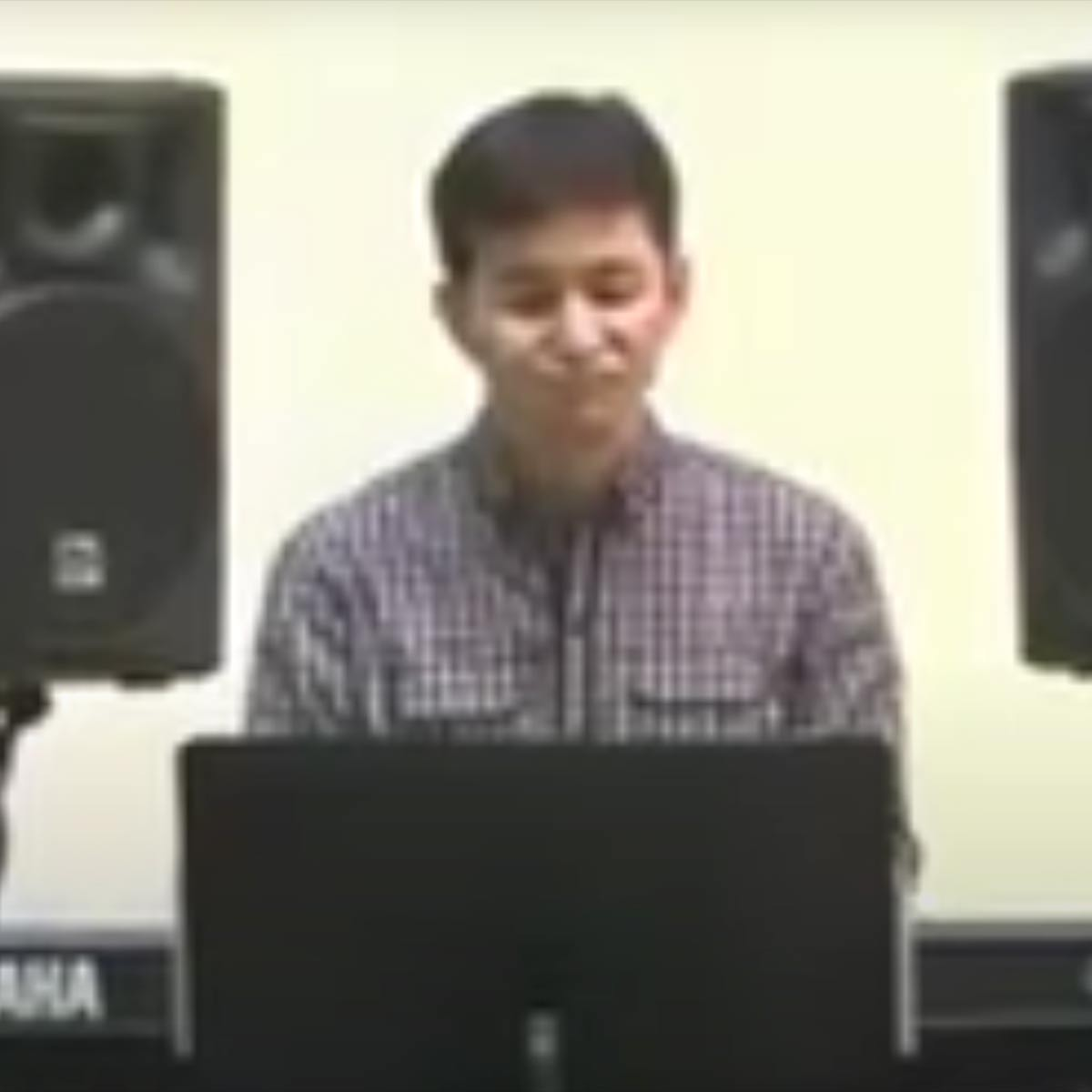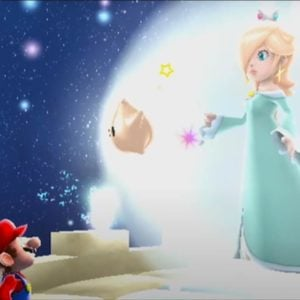# Mahito Yokota

3 Music Sheets

• ###### Birth Name:

Mahito Yokota

• ###### Genres:

Mahito Yokota (Yokota Mahito) is a Japanese music composer and orchestrator who works for the video game development company Nintendo. He is most known for his collaborations with Koji Kondo in the Super Mario Galaxy series, along with Super Mario 3D World. Prior to joining Nintendo in 2003, Yokota served as an audio director at Koei.Credit: Wikipedia

## Artist's Music Sheets

•T O s S g H T O s S g H T O s S g H T O s S g H [cT] O s S g H T O s [ZS] [gc] [ZH] [TL] O [sl] S g [LH] [lT] O [sJ] S g [gH] [tH] O s D g H t O s D g H [tD] O s D g H t O s D g H [cT] O s S g H T O s [ZS] [gc] [vH] [VT] O s [vS] [gc] [ZH] [TL] O [sZ] S g [LH] [tl] O [sZ] D H l t O s D H l t O s D H [lZ] t O [sH] t [ZO] D [uZ] a S D G k u a S [LD] [kG] J [uk] a S D G k u a S [kD] [uL] [aZ] [yx] p [jd] f g j y p d f g j [yc] p d [jf] g j L j g [jd] [pk] [li] [uL] a S D G k u a S D [kG] L [uZ] a [SL] D [kG] k [uJ] a [kS] D [uL] [aZ] [yx] p [jd] f g j y p d f g j [zy]cbp d [jf]zcg j [lj]||[sH]||T O s S g H T O s S g H T O s S g H T O s S g H [cT] O s S g H T O s [ZS] [gc] [ZH] [TL] O [sl] S g [LH] [lT] O [sJ] S g [gH] [tH] O s D g H t O s D g H [tD] O s D g H t O s D g H [cT] O s S g H T O s [ZS] [gc] [vH] [VT] O s [vS] [gc] [ZH] [TL] O [sZ] S g [LH] [tl] O [sZ] D H l t O s D H l t O s D H [lZ] t O [sH] t [ZO] D [uZ] a S D G k u a S [LD] [kG] J [uk] a S D G k u a S [kD] [uL] [aZ] [yx] p [jd] f g j y p d f g j [yc] p d [jf] g j L j g [jd] [pk] [li] [uL] a S D G k u a S D [kG] L [uZ] a [SL] D [kG] k [uJ] a [kS] D [uL] [aZ] [yx] p [jd] f g j y p d f g j [zy]cbp d [jf]zcg j [lj]||[sH]

Level: 5
Length: 01:57
Intermediate

#### Mahito Yokota

•[f6] T T G T T h T T j T T [f6] T T G T T h T T j| [I2]p[G9] 9S[f\$] [dQ] QP[S5] [wa] [wd] u w w [d6] [eS] eod [eS] eo[a2] [yi] [ya] p y y [I9]p[yG] yS[fQ] [dI] Ip[wa]d[oj] od[jE] [hP] P [he] [pG] pd[eG] [pf] pS[yd9] y y y| [I2]p[G9] 9S[f\$] [dQ] QP[S5] [wa] [wd] u w w [d6] [eS] eod [eS] eo[a2] [yi] [ya] p y y [I9]p[yG] yS[fQ] [dI] Ip[wa]d[oj] od[jE] [hP] P [he] [pG] pd[eG] [pf] pS[yd9] y y y| [wj] d f j f L [jQ]| d| [j0] d f j f L [j9]| x L| [j5] d f j f L [j\$]| d| [a3] r r S r r [d6] r r f| [I2]p[G9] 9S[f\$] [dQ] QP[S5] [wa] [wd] u w w [d6] [eS] eod [eS] eo[a2] [yi] [ya] p y y [I9]p[yG] yS[fQ] [dI] Ip[wa]d[oj] od[jE] [hP] P [he] [pG] pd[eG] [pf] pS[yd9] y y y| [f6] T T G T T h T T j T T [f6] T T G T T h T T j| [I2]p[G9] 9S[f\$] [dQ] QP[S5] [wa] [wd] u w w [d6] [eS] eod [eS] eo[a2] [yi] [ya] p y y [I9]p[yG] yS[fQ] [dI] Ip[wa]d[oj] od[jE] [hP] P [he] [pG] pd[eG] [pf] pS[yd9] y y y| [wj] d f j f L [jQ]| d| [j0] d f j f L [j9]| x L| [j5] d f j f L [j\$]| d| [a3] r r S r r [d6] r r f| [I2]p[G9] 9S[f\$] [dQ] QP[S5] [wa] [wd] u w w [d6] [eS] eod [eS] eo[a2] [yi] [ya] p y y [I9]p[yG] yS[fQ] [dI] Ip[wa]d[oj] od[jE] [hP] P [he] [pG] pd[eG] [pf] pS[yd9] y y y

Level: 5
Length: 02:13
Intermediate

#### Mahito Yokota

•[8t] [9y] [8t] [wo] [wo] [ep] [EP] [ig] [uf] [yd] [ts] t r y t w 0 q w w q y T u y t r e r e w o I p o i u i u o i u y W u Y i u y T u y t r t y [ts] [ra] [yd] [ts] [wo] [0u] [qi] [wo] [wo] [QI] [yd] [TS] [uf] [yd] [ts] [EP] [WO] [EP] [WO] [wo] o p P a s d f g [odh] [ISG] [pFj] [odh] [iSg] [upf] [idg] [uSf] [ofh] [idh] [usf] [yOd] O [uof] [yod] [iog] [uof] [yod] [WyO] [EyP] [tuos] t t i T i O i O o t t i T i O P O O o o o s P O P o Y Y Y O o i o Y Y Y i o y o [ra] [wo] [yd] [idg] [yd] [EyP] [yPd] [iPg] [PdJ] [yidgz] y t w y t w u y y u i o o p u u y u i o i u t u y [wo] [yd] [ts] [wo] [yd] [ts] [wo] [uf] [yd] [yad] [ahk] [pgj] [ofh] [ofh] [pgj] [PhJ] [pgj] [ofh] [uSf] [idg] [idg] [ofh] [OgH] [ofh] [OgH] [JhJ] [sfhl]
Level: 6
Intermediate